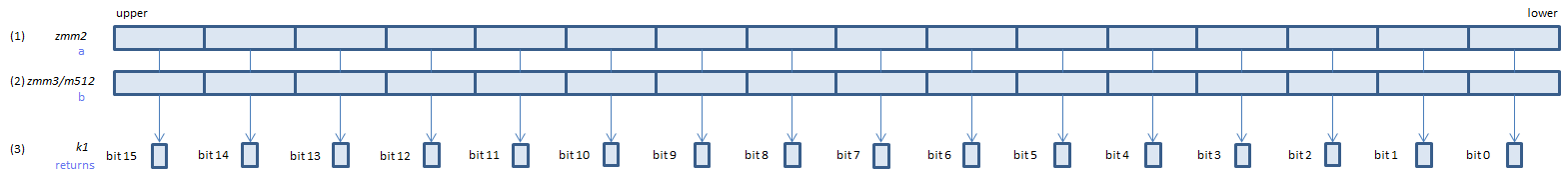﻿ vcmpneq_usps

## VCMPNEQ_USPS - CoMPare Not EQual Unordered Signal Packed Single

VCMPNEQ_USPS xmm1, xmm2, xmm3/m128    (V1
__m128 _mm_cmp_ps(__m128 a, __m128 b, _CMP_NEQ_US)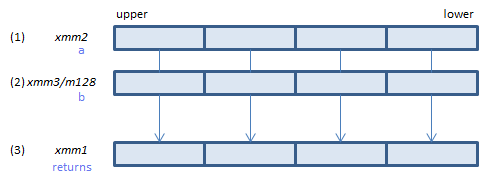For each float, if (1) != (2) or either or both of (1)(2) is NaN, set 1, else set 0, to all bits of the corresponding float of (3).
VCMPNEQ_USPS ymm1, ymm2, ymm3/m256    (V1
__m256 _mm256_cmp_ps(__m256 a, __m256 b, _CMP_NEQ_US)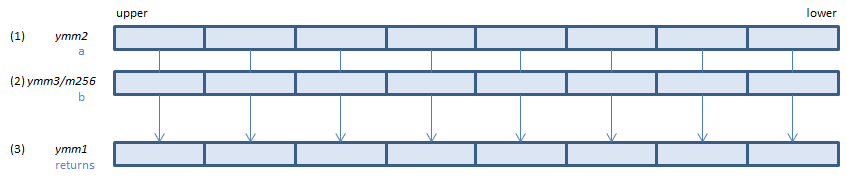For each float, if (1) != (2) or either or both of (1)(2) is NaN, set 1, else set 0, to all bits of the corresponding float of (3).
VCMPNEQ_USPS k1{k2}, xmm2, xmm3/m128/m32bcst    (V5+VL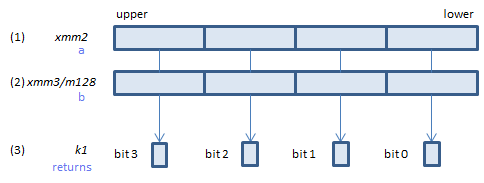For each float, if (1) != (2) or either or both of (1)(2) is NaN, set 1, else set 0, to the corresponding bit of (3).
If k2 bit is 0, the comparison is not done and the corresponding bit of (3) is set to zero. Upper bits of (3) are zero cleared.
VCMPNEQ_USPS k1{k2}, ymm2, ymm3/m256/m32bcst    (V5+VL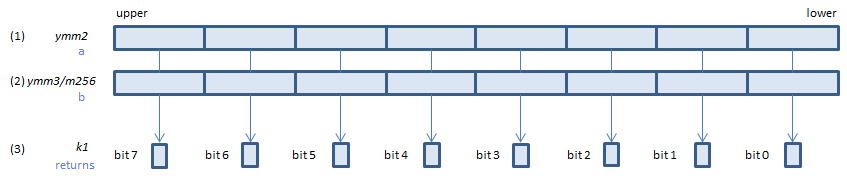For each float, if (1) != (2) or either or both of (1)(2) is NaN, set 1, else set 0, to the corresponding bit of (3).
If k2 bit is 0, the comparison is not done and the corresponding bit of (3) is set to zero. Upper bits of (3) are zero cleared.
VCMPNEQ_USPS k1{k2}, zmm2, zmm3/m512/m32bcst{sae}    (V5